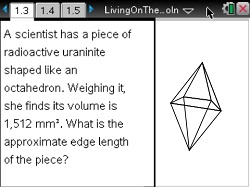••• ##### Device
•TI-Nspire™ CX/CX II
•TI-Nspire™ CX CAS/CX II CAS
• TI-Nspire™ Navigator™
• TI-Nspire™
• TI-Nspire™ CAS
• ##### Software

TI-Nspire™
TI-Nspire™ CAS

3.0

• ##### Report an Issue

Algebra 2: Living on the Edge
by Texas Instruments

#### Overview

Students build a solution to a rather complex problem: Finding the edge length of an octahedron given its volume by solving two simpler problems first.

#### Key Steps

•Students build a solution to a rather complex problem—finding the edge length of an octahedron given its volume—by solving two simpler problems.

•First, students find a formula for the edge length of a square given its area.

•Students write an equivalent formula with fractional exponents and substitute values to find the edge length of a particular square.